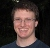# Profile Likelihood CI for mediated effect

3 posts / 0 newOffline
Joined: 10/12/2009 - 14:55
Profile Likelihood CI for mediated effect

Hello,

I am relatively new to OpenMx. I am wondering if it's possible to compute a profile likelihood CI using OpenMx. I saw the documentation for mxCI(), but I would appreciate if one can provide OpenMx code to estimate a profile Likelihood CI for mediated effect in single mediator model with all observed variable.

I also saw Pek, J. & Wu, H. (2015) article, but it appears that mxCI() can implement their approach, if I am correct.

DavoodOffline
Joined: 03/01/2013 - 11:03
Yep

Hi Davood,

It looks like we may need to improve the documentation for mxCI(). All the confidence intervals provided by OpenMx are profile likelihood CIs. Moreover, I believe in the last year we have updated how the profile likelihood CIs were done to match Hao Wu's work.

Here's an example of a multiple regression using mxPath() statements: https://github.com/OpenMx/OpenMx/blob/master/inst/models/passing/IntroSEM-MultivariateRegRaw.R . It could be modified without too much trouble to do mediation. A profile likelihood CI could then be added as demonstrated in the example on the help page for mxCI(): create an mxCI() object that refers to the free parameters or other named entities on which you want the CI, put this object in the model, run the model using mxRun() with intervals=TRUE, then look at the results using summary().Offline
Joined: 10/08/2009 - 22:37
Hi Davood,Here is an example

Hi Davood,

Here is an example if you are new to OpenMx.

Mike

library(OpenMx)
set.seed(123456)
n <- 200

## Generate some simulated data
x <- rnorm(n)
m <- 0.5*x+rnorm(n)
y <- 0.2*x+0.5*m+rnorm(n)

my.df <- data.frame(y, m, x)
dataRaw <- mxData( observed=my.df, type="raw" )

## Model
A <- mxMatrix(type = "Full", values = 0,
nrow=3, ncol=3,
free = c(FALSE,TRUE,TRUE,
FALSE,FALSE,TRUE,
FALSE,FALSE,FALSE),
labels = c(NA,"b","c",
NA,NA,"a",
NA,NA,NA),
byrow = TRUE, name = "A")

S <- mxMatrix(type = "Diag",
values = c(1,1,1),
nrow=3, ncol=3,
free = c(TRUE,TRUE,FALSE),
labels = c("ErrVar_y", "ErrVar_m", "Var_x"),
name = "S")

M <- mxMatrix(type = "Full",
nrow=1, ncol=3,
values = 0,
free = TRUE,
labels = c("inter_y", "inter_m", "mean_x"),
name = "M")

F <- mxMatrix(type = "Iden",
nrow=3, ncol=3,
name = "F")

## Indirect effect
ind <- mxAlgebra(a*b, name="ind")

exp <- mxExpectationRAM("A","S","F","M",
dimnames=c("y", "m", "x"))
funML        <- mxFitFunctionML()

indirect <- mxModel("Indirect effect", dataRaw,
A, S, M, F, ind, exp, funML,
mxCI("ind"))

indirect.fit <- mxRun(indirect, intervals=TRUE)
summary(indirect.fit)